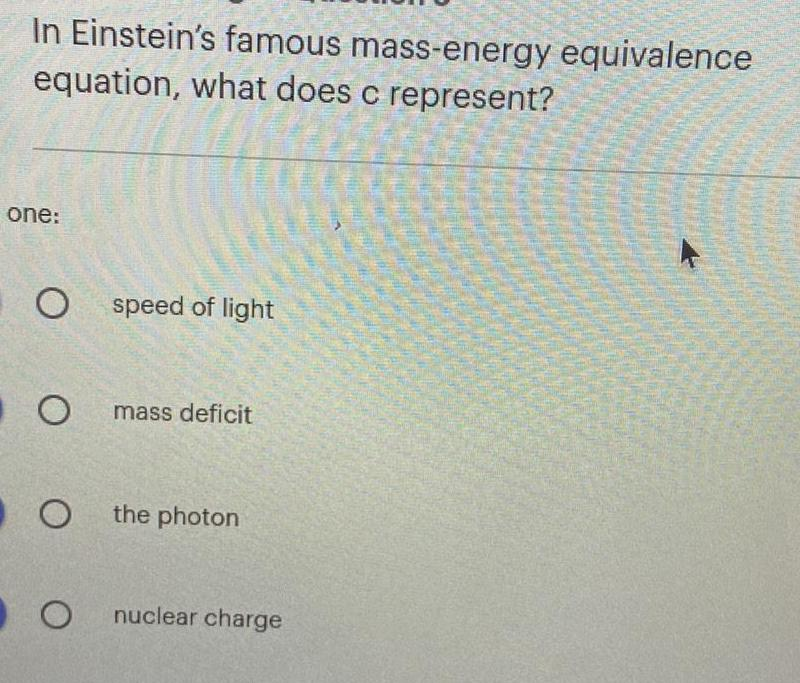Question:

# In Einstein's famous mass-energy equivalence equation, what

Last updated: 8/9/2022In Einstein's famous mass-energy equivalence equation, what does c represent? speed of light mass deficit the photon nuclear charge